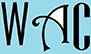## Divisibility Rule: Math Games for Class and Play

Purpose: To make you comfortable with signs when you add and subtract.

A teacher or an knowledgeable adult may make students form a circle and ask them questions in turns, and whoever gets the answer wrong leaves the circle.  The winner is the last student in the circle.

A teacher or an knowledgeable adult may make students form two or more teams of equal members and ask questions in turns.  When a team gets it right, they get 2 points, but if they get it wrong, they lose 1 point.  If Team A gets it wrong, they lose a point and Team B gets a chance to get 1 point.  If Team B misses the opportunity, they do not lose 1 point because it was not their question originally, then Team C gets the chance to gain the extra point.  The teacher or adult can then answer the question with explanations if all the teams missed it.  Team A loses 1 point, no other team loses a point, and Team B gets the next question.

Game Items: Pencils, pens, and papers are allowed, but each question must be answered within 5 seconds after the teacher or adult finishes the question.  A time keeper and a score keeper may be required to assist the teacher or adult.  The game is good for classrooms, parks, picnic, field trips, on the bus, parties, or family time.

Reward for winners: Extra cookie or scope of ice cream at picnics, first to get in and out of the bus on field trips (seat anywhere), special treatment to any game at the park, early lunch, exemption from time-out or non-academic work for the day in class, and exemption from cleaning dishes or extended video game or movie time at home.

The game is best for teachers and parents who have explained to their children the 10 rules of divisibility.  This is how to figure out which number can divide what.  Let us find out if 48 can be divided by numbers 1-10.

Some Questions:

Can 48 be divided by 1? =  Yes, because 1 can divide every number

Can 48 be divided by 2? =  Yes, because 2 can divide every number that ends with an even number like 8

Can 48 be divided by 3? =  Yes, because 3 can divide every number whose sum of their digits is divisible by 3 (4 + 8 = 12 and 3 can divide 12)

Can 48 be divided by 4? =  Yes, because 2 can divide the number twice (The last digit is even so 2 can divide it, 48/2 = 24 and 24/2 = 12)

Can 48 be divided by 5? =  No, because 5 cannot divide any number that does not have a last digit of 0 or 5

Can 48 be divided by 6? =  Yes, because 2 and 3 can divide the number (it ends with an even number so 2 will divide it and if you add the digits 3 will divide it)

Can 48 be divided by 7? =  Just do the math, 48/7 = not possible.

Can 48 be divided by 8? =  Yes, because 2 and 4 can divide it (it ends with an even numbers and 2 will divide it twice like above).

Can 48 be divided by 9? =  No, because 3 cannot divide the number twice (The sum of the number is divisible by 3, 4 + 8 = 12. 48/3 = 16, but 1 + 6 = 7, so 9 will not divide it)

Can 48 be divided by 10? =  No, because 10 cannot divide any number that does not have a last digit of 0